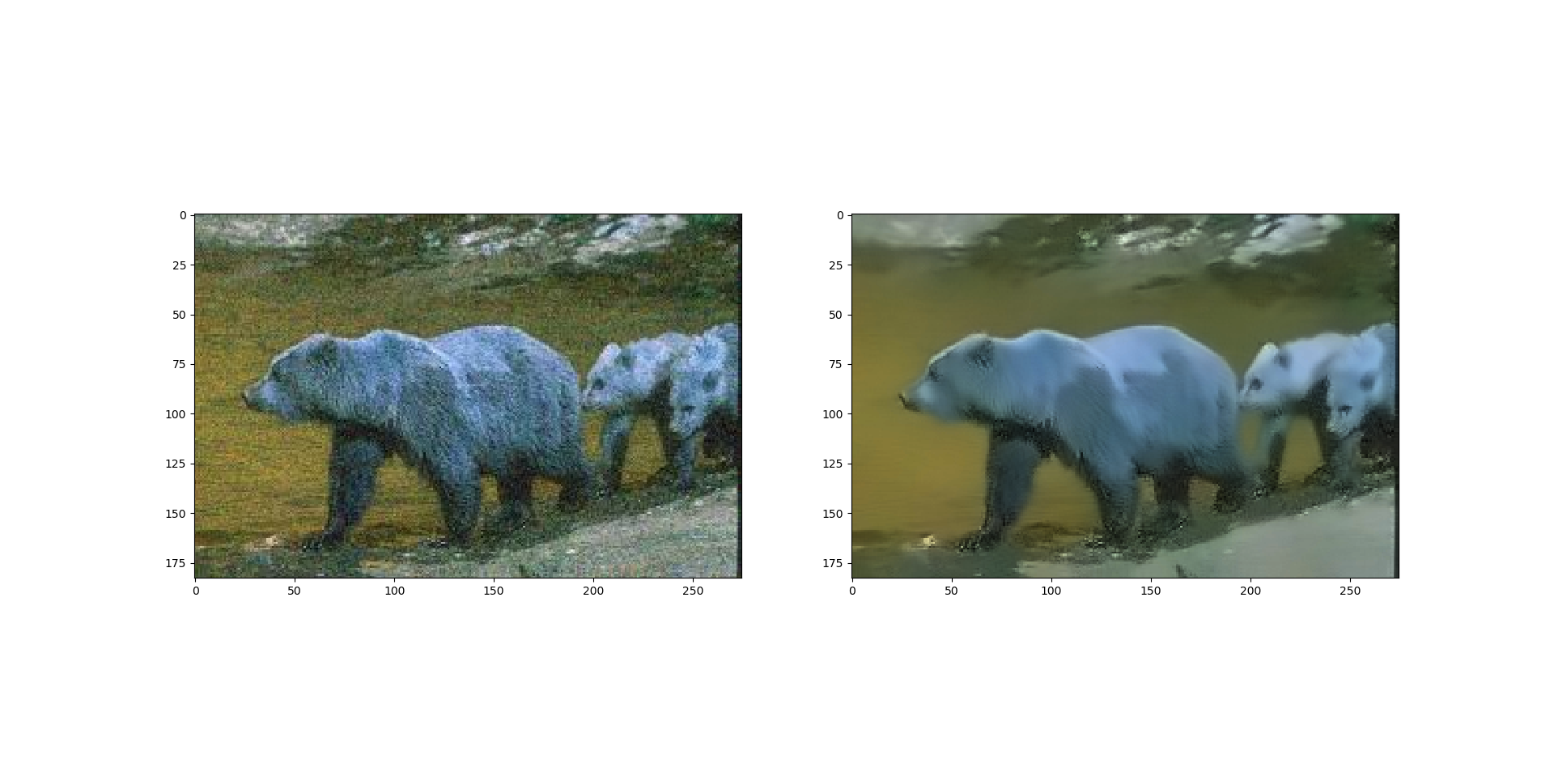Open In App
Related Articles

# Python | Denoising of colored images using opencv

Denoising of an image refers to the process of reconstruction of a signal from noisy images. Denoising is done to remove unwanted noise from image to analyze it in better form. It refers to one of the major pre-processing steps. There are four functions in opencv which is used for denoising of different images.

Syntax: cv2.fastNlMeansDenoisingColored( P1, P2, float P3, float P4, int P5, int P6)

Parameters:
P1 – Source Image Array
P2 – Destination Image Array
P3 – Size in pixels of the template patch that is used to compute weights.
P4 – Size in pixels of the window that is used to compute a weighted average for the given pixel.
P5 – Parameter regulating filter strength for luminance component.
P6 – Same as above but for color components // Not used in a grayscale image.

Below is the implementation:

 `# importing libraries``import` `numpy as np``import` `cv2``from` `matplotlib ``import` `pyplot as plt`` ` `# Reading image from folder where it is stored``img ``=` `cv2.imread(``'bear.png'``)`` ` `# denoising of image saving it into dst image``dst ``=` `cv2.fastNlMeansDenoisingColored(img, ``None``, ``10``, ``10``, ``7``, ``15``)`` ` `# Plotting of source and destination image``plt.subplot(``121``), plt.imshow(img)``plt.subplot(``122``), plt.imshow(dst)`` ` `plt.show()`

Output: